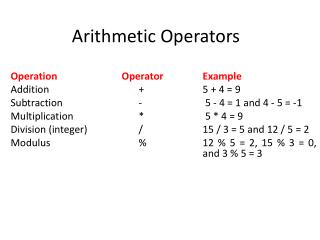Download PresentationArithmetic Operators

Loading in 2 Seconds...

# Arithmetic Operators - PowerPoint PPT Presentation

Arithmetic Operators. Operation Operator Example Addition + 5 + 4 = 9 Subtraction - 5 - 4 = 1 and 4 - 5 = -1 Multiplication * 5 * 4 = 9 Division (integer) / 15 / 3 = 5 and 12 / 5 = 2 Modulus % 12 % 5 = 2, 15 % 3 = 0, and 3 % 5 = 3.I am the owner, or an agent authorized to act on behalf of the owner, of the copyrighted work described.
Download Presentation## Arithmetic Operators

An Image/Link below is provided (as is) to download presentation

Download Policy: Content on the Website is provided to you AS IS for your information and personal use and may not be sold / licensed / shared on other websites without getting consent from its author.While downloading, if for some reason you are not able to download a presentation, the publisher may have deleted the file from their server.

- - - - - - - - - - - - - - - - - - - - - - - - - - E N D - - - - - - - - - - - - - - - - - - - - - - - - - -
Presentation Transcript
1. Arithmetic Operators Operation Operator Example Addition + 5 + 4 = 9 Subtraction - 5 - 4 = 1 and 4 - 5 = -1 Multiplication * 5 * 4 = 9 Division (integer) / 15 / 3 = 5 and 12 / 5 = 2 Modulus % 12 % 5 = 2, 15 % 3 = 0, and 3 % 5 = 3

2. Fundamental C++ Variables

3. (Sum of digits) Make a program that reads a three-digit and then calculates sum of the digits. (Hint: Use the modulus (%) and integer division (/) operators.)

4. #include <iostream> using namespace std; int main() { int num,a,b,c,sum; cout<<"Enter 3 digit integers"<<endl; cin >> num; a=(num%10); b=(num %100)/10; c=num/100; sum = a+b+c; cout <<a<<“+”<<b<<“+”<<c<<“=“<<sum<<endl; system("PAUSE"); return 0; }

5. Using Text Files as Input and Output C++ provides two functions to read from a text file and to write into a text file. Those functions are ifstream() and ofstream(). Both functions are declared in the <fstream> header. ifstreamopens an existing input file whereas, ofstreamcreates or recreates and opens the output file. After you have finished with the input and output files you should close them so that their resources become available again for the system. close() function is used to close the open files.

6. The following program read two integers (num1 and num2) from the file numbers.in. Computes sum, difference, product and quotient of those two numbers and then writes the result into the file numbers.out #include <fstream> using namespace std; int main() { ifstreamfin("numbers.in"); //open input file ofstreamfout("numbers.out");//create and open output file intnum1, num2; fin >>num1 >>num2; //read two integers from the input file. fout <<"sum is "<<num1+num2<<endl; fout<<"difference is "<<num1-num2<<endl; fout<<"product is "<<num1*num2<<endl; fout <<"integer quotient is "<<num1/num2<<endl; fout<<"floating-point quotient is "<<(float)num1/num2<<endl; fin.close(); //close the input file fout.close(); //close the output file system("PAUSE"); return 0; }

7. HOMEWORK (Sum of digits) Make a program that reads a four-digit integer from the file "number.in" and then calculates sum of the digits and writes the result into the file "sum.out". (Hint: Use the modulus (%) and integer division (/) operators.)